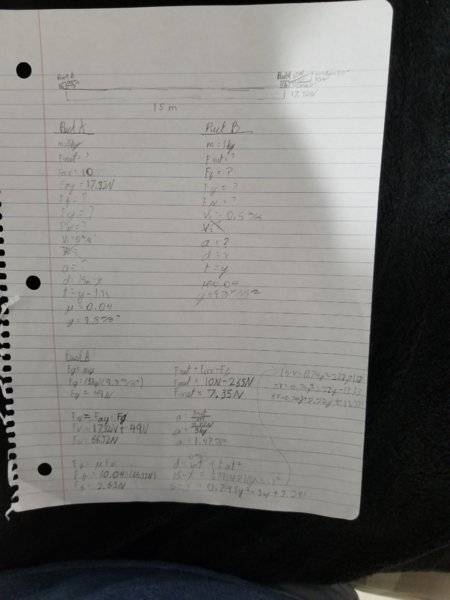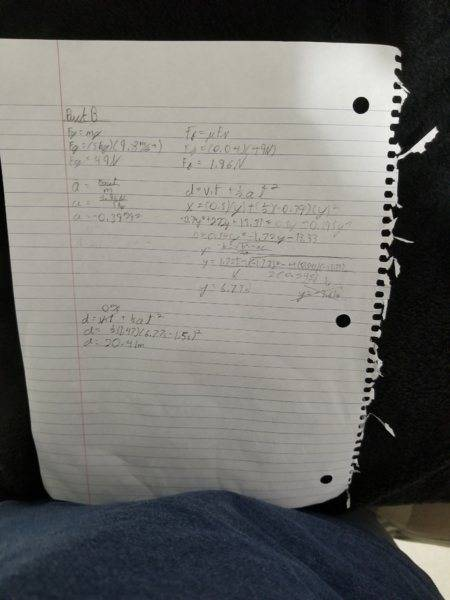# Complex Kinematics and Dynamics

## Homework Statement

Two pucks (5 kg each) made of Teflon are on a long table, also made of Teflon. Puck A is sitting at
rest on the left end of the table. Puck B is 15 m away at the right hand end of the table, and is
travelling toward Puck A with an initial speed of 0.5 m/s. A person on the left waits 1.5 seconds and
then pushes Puck A forward from rest with a force of 20 N applied at an angle of 30° to the horizontal.
If that force is maintained at a steady rate for the entire question, how far will puck A travel before it
strikes puck B? (The coefficient of friction between the Teflon and Teflon is 0.04.

2. The attempt at a solution:

Puck A
Fax = 20NSin30
Fax = 10N

Fay = 20NCos30
Fay = 17.32N

Fg = mg
Fg = (5kg)(9.8m/s^2)
Fg = 49N

Fn = Fay + Fg
Fn =17.32N + 49N
Fn = 66.32

Ff = uFn
Ff = (0.04)(66.32N)
Ff = 2.65

Fnet = Fax - Ff
Fnet = 10N - 2.65
Fnet = 7.35N

a = Fnet/m
a = 7.35N/5kg
a = 1.47m/s^2

d = v1t + 1/2at^2
15-x = 1/2(1.47)(y-1.5)^24
x = -0.74y^2 + 2.22y +13.33

Puck B
Fg = mg
Fg = (5kg)(9.8m/s^2)
Fg = 49N

Ff = uFn
Ff = (0.04)(49N)
Ff = 1.96N

Since there is no applied force Fnet = -Ff

a = Fnet/m
a = -1.96/5kg
a = -0.39m/s^2

d = v1t + 1/2at^2
x = (0.5)(y) + 1/2(-0.39)(y)^2
-0.74y^2 + 2.22y +13.33 = 0.5 - 0.195y^2
0 = 0.545y^2 - 1.72y-13.33

I then put it in the quadratic formula and got:
y = 6.77s and y = -3.61s(omited)

Puck A Distance
d = v1t + 1/2at^2
d = (0m/s)(6.77s-1.5s) + 1/2(1.47m/s^2)(6.77-1.5s)^2
d = 20.41m(This answer doesn't make sense)#### Attachments

Last edited:

Doc Al
Mentor

gneill
Mentor
Your images are on your private google drive, so the links are inaccessible to other members. Either type out your solutions as suggested, or at least use the UPLOAD facility to make the images local to the PF server.

I uploaded the photos from a local source, so you should be able to see my work. Thank you for taking the time to help me.

Your images are on your private google drive, so the links are inaccessible to other members. Either type out your solutions as suggested, or at least use the UPLOAD facility to make the images local to the PF server.
I uploaded the photos from a local source, so you should be able to see my work. Thank you for taking the time to help me.

gneill
Mentor
I uploaded the photos from a local source, so you should be able to see my work. Thank you for taking the time to help me.